# Work and Energy class 9 notes

Welcome to Class 9 Work and energy Notes for Chapter 11.The topics in this page are Introduction to Work and energy ,What is Work in Physics,positive,negative work, Kinetic energy and Potential.This is according to CBSE and the NCERT textbook. If you like the study material, feel free to share the link as much as possible.

Table of Content

## Introduction to work and energy

• In our everyday life we use terms like work and energy.
• Term work is generally used in context to any kind of activity requiring physical or mental effort.
• When we push or pull a heavy load or lift it above the floor then we are doing work, but a man carrying heavy load and standing still is not doing any work according to scientific definition of work.
• Another term we often use is energy. Energy is usually associated with work done in the sense that a person feeling very energetic is capable of doing lot of work.
• This way energy is defined to be as capacity of doing work.
• There are many forms of energy like chemical energy, mechanical energy, electrical energy, heat energy etc. These forms of energies can be used in number of ways.
• One form of energy can be converted into another form of energy.
• In this chapter we will study about work, relation between work and energy, conservation of energy etc.

## What is Work in Physics

• We already know that work is said to be done when a force produces motion.
• So we can formally define work done as
Work done by force acting on an object is equal to the product of force and the displacement of the object in the direction of the force.
• Work done is defined in such a way that it involves both force applied on the body and the displacement of the body.
• Consider the figure given below where a block is placed on a frictionless horizontal floor. This block is acted upon by a constant force F. Action of this force is to move the body through a distance d in a straight line in the direction of force.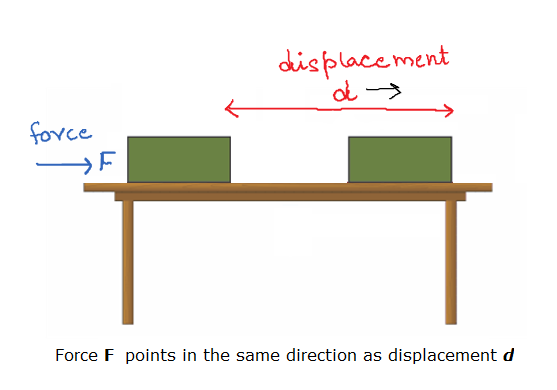• Now, work done by this force is equal to the product of the magnitude of applied force and the distance through which the body moves. Mathematically,
W=Fd
• Here both force and displacement have magnitude as well as directions. So both of these quantities are vectors. Work, which is the product of force and displacement, has only magnitude and no direction. So it is a scalar quantity.
• If F = 1 N and d = 1 m then the work done by the force will be 1 N m.
• Here the unit of work is Newton meter (N m) or joule (J). Thus 1 J is the amount of work done on an object when a force of 1 N displaces it by 1 m along the line of action of the force.
• The work done by a force can be either positive or negative or zero.

### Positive Work

•  If a force displaces the object in its direction, then the work done is positive
So, W=Fd
The example of this kind of work done is motion of ball falling towards ground where displacement of ball is in the direction of force of gravity.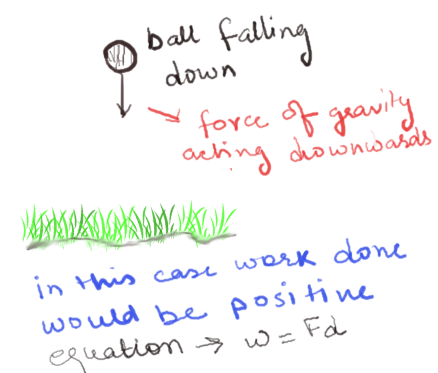• ### Negative work

• If the force and the displacement are in opposite directions, then the work is said to be negative. For example if a ball is thrown in upwards direction, its displacement would be in upwards direction but the force due to earth’s gravity is in the downward direction.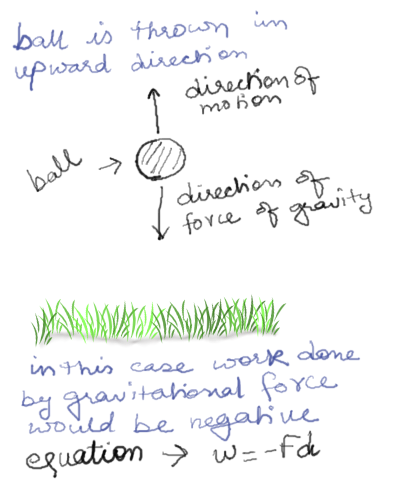So here in this case gravity is doing negative work when you throw the ball upwards. Hence the work done by gravitational force is negative. Mathematically when displacement is opposite to the force work done is given by
• Negative work just means that the force and the displacement act in opposite directions.

### Case of zero work done

• If the directions of force and the displacement are perpendicular to each other, the work done by the force on the object is zero.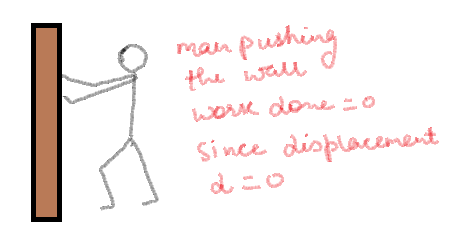For example, when we push hard against a wall, the force we are exerting on the wall does no work, because in this case the displacement of the wall is d = 0. However, in this process, our muscles are using our internal energy and as a result we get tired.

### Displacement at an angle to the force

• To understand this let us consider a block of mass m sliding on an inclined surface as shown below in the figure.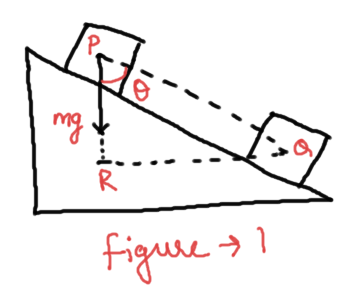• Now the object moves along the inclined surface from point P to Q. So its displacement would be PQ. The force acting on this block due to force of gravity would be
$F = mg$
which acts in vertically downwards direction.
• Now here our problem is to find the work done by the gravitational force in moving the block from point P to point Q.
• When the block moves from point P to Q, it comes down through a height PR. So here we can also say that the object is displaced in the vertically downwards direction through a distance PR and at the same time it is also displaced horizontally through a distance RQ. Since the displacement in the direction of force is PR , the work done would be
$W = mg(PR) = mg(PQcos\theta )$
• If displacement d of any object makes an angle $\theta$ with the force $F$ acting on it as shown below in the figure , then the work done by the force is
$W = Fd\cos \theta$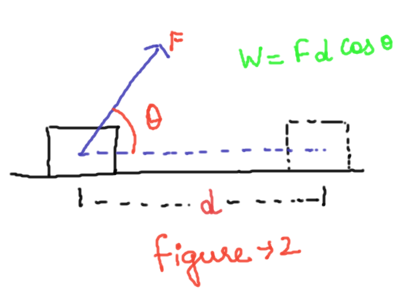• Now again consider the figure 1 where $PQ$ is the actual displacement but here you must note that $PQcos\theta$ is the displacement of the block in the direction of force which is along $PR$. So we can say that $PQcos\theta$ is the component of displacement $PQ$ along $PR$.
• So, general equation of work done would be
Work=force × component of displacement along the direction of force

## Energy

• We already have an idea that energy is associated closely with work and we have defined energy of a body as the capacity of the body to do work. So, an object having a capability to do work is said to possess energy.
Definition: - The capacity of an object to do work is called energy of the object.
• The object which does the work loses energy and the object on which the work is done gains energy.
• An object that possesses energy can exert a force on another object. When this happens, energy is transferred from the former to the latter.
• The energy possessed by an object is measured in terms of its capacity of doing work.
• The unit of energy is, therefore, the same as that of work, that is, joule (J). 1 J is the energy required to do 1 joule of work.
• There are various forms of energy available to us for example mechanical energy (potential energy + kinetic energy), heat energy, chemical energy, electrical energy and light energy.

### Kinetic Energy

• Kinetic energy is the energy possessed by the body by virtue of its motion
• Body moving with greater velocity would posses greater K.E in comparison of the body moving with slower velocity
• Kinetic energy of a body moving with a certain velocity is equal to the work done on it to make it acquire that velocity.
• Consider a body of mass m moving under the influence of constant force F. From Newton’s second law of motion
$F=ma$
Where a is the acceleration of the body
• If due to this acceleration a, velocity of the body increases from${v_1}$ to${v_2}$ during the displacement d then from equation of motion with constant acceleration we have
$v_2^2 - v_1^2 = 2ad$
Or,
$a = \frac{{(v_2^2 - v_1^2)}}{{2d}}$
Using this acceleration in Newton's second law of motion
we have
$F = \frac{{m\left( {v_2^2 - v_1^2} \right)}}{{2d}}$
or
$Fd = \frac{{m\left( {v_2^2 - v_1^2} \right)}}{2}$
or
$Fd = {{mv_2^2} \over 2} - {{mv_1^2} \over 2}$
We know that Fd is the work done by the force F in moving body through distance d.
• In above equation, quantity on the right hand side $\frac{1}{2}m{v^2}$ is called the kinetic energy of the body
• Thus kinetic energy possessed by an object of mass m and moving with uniform velocity v is
${E_K} = \frac{1}{2}m{v^2}$
• If the mass of the body is doubled, its kinetic energy also gets doubled and if mass of the body is halved its kinetic energy also gets halved.
• If the velocity of the body is doubled its kinetic energy becomes four times and if the velocity of the body id halved, then its kinetic energy becomes one fourth.

### Potential Energy

• Potential energy is the energy stored in the body or a system by virtue of its position in field of force or by its configuration.
• Force acting on a body or system can change its Potential Energy.
• Few examples of bodies possessing PE are given below
i) Stretched or compressed coiled spring
ii) Water stored up at a height in the Dam possess PE
iii) Any object placed above the height H from the surface of the earth posses PE
• Potential energy is denoted by letter U.
• All bodies fall towards the earth with a constant acceleration known as acceleration due to gravity.
• Consider a body of mass m placed at height h above the surface of the earth as shown below in the figure.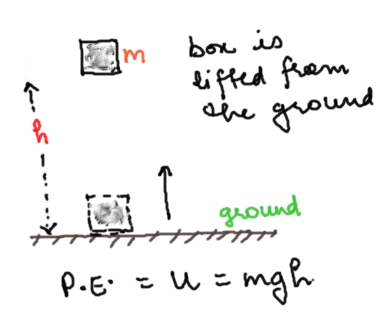• The force acting on this body is the gravitational pull of the earth m×g which acts in the downwards direction.
• To lift the body above the surface of the earth, we have to do work against the force of gravity. Now, Work Done= Force × distance = m×g×h
Where,
m= mass of the body;
g= acceleration due to gravity;
h= height of the body above the reference point, say the surface of earth.
• Since work done on the object is equal to $mgh$, an energy equal to $mgh$ is gained by the object. ${E_p} = mgh$
Here from the above equation we see that potential energy of any object at a height depends on the ground level or the zero level you choose.
• One important point to note hare is that work done by gravity depends on the difference in vertical heights of the initial and final positions of the object and not on the path along which the object is moved.
• To understand this point consider the figure given below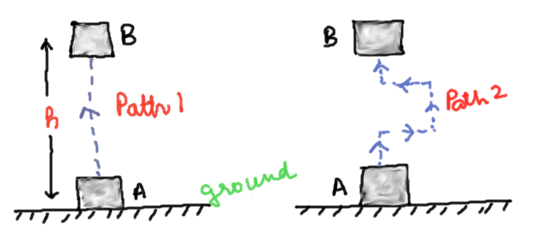Now this figure shows the block is raised from position A to position B through two different paths. If $h$ is the height then in both the situations work done on the object is $mgh$.

## Summary

Here is Work and energy Class 9 Notes Summary
• Work done is defined as the magnitude of the force multiplied by the distance moved by the object in the direction of the applied force. The unit of work is joule: 1 joule = 1 newton x 1 metre.
• An object having capability to do work is said to possess energy. Energy has the same unit as that of work.
• An object in motion possesses what is known as the kinetic energy of the object. $KE=\frac {1}{2} mv^2$
• The energy possessed by a body due to its change in position or shape is called the potential energy. The gravitational potential energy =$mgH$
• Law of conservation of energy states energy can only be transformed from one form to another; it can neither be created nor destroyed. The total energy before and after the transformation always remains constant.
• Power is defined as the rate of doing work. The SI unit of power is watt. 1 W = 1 J/s.

• Notes
• Assignment
• NCERT Solutions